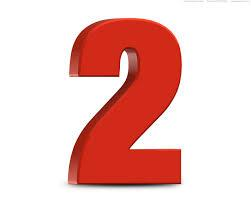# Multiply 2700

I think the number. If I add five and multiply the result by 7, I get 245. Which is it?

x =  30

### Step-by-step explanation:Did you find an error or inaccuracy? Feel free to write us. Thank you!

Tips for related online calculators
Do you have a linear equation or system of equations and looking for its solution? Or do you have a quadratic equation?